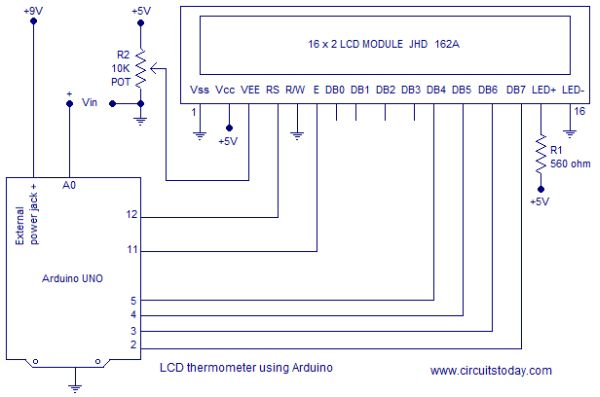# Voltmeter using arduino

## 0-5V voltmeter using arduino.

This article is about a simple 3 digit voltmeter using arduino. The circuit can measure anything between 0 to 5V at an accuracy of 50mV. The circuit uses minimum number of external components and can be easily modified for different voltage ranges. The display device is a common anode multiplexed seven segment LED display module (Type No:E1-3056ASR1). Let’s have a look at the display device first.## E1-3056ASR1.

It is a low cost multiplexed 3 digit seven segment LED module of common anode type. Using such a multiplexed module instead of three separate display modules save a lot of wiring and effort. Such multiplexed modules of different configurations are now commonly available in the market. The figure below shows  pin out of the E1-3056ASR1 display module used here.

Read this article Voltmeter using  8051 for a better grasp. The idea is same  but instead of an 8051 microcontroller Arduino-uno is used here. Circuit diagram of the voltmeter using arduino is shown in the figure below.

## Circuit diagram.

Common anode terminals of each display are interfaced to the digital output pins 1, 2, 3 of the arduino respectively. Segments a to dotpoint of the display are interfaced to the digital output pins (5 to 12) of the arduino. The voltage to be measured is applied to the analog input pin A0 of the arduino. Arduino-Uno has six analog input channels named A0, A1, A2, A3, A4 and A5. The voltage in the range of 0 to 5V applied to each of the pin can be converted into a digital value in the range 0 to 1023 using “analogRead” function. That means the sensitivity of each internal ADC channel is 5/1023 which  is equal to 4.88mV. The program for  digital voltmeter using arduino is shown below.

READ  Digital Arduino Voltmeter with Temperature

For more detail:Voltmeter using arduino

#### This Post / Project can also be found using search terms:

• e1-3056asr1 pins
• E1-3056asr1 seven segment
• how 3 digit led voltmeter circuit using arduino works